Multiplication of Fractions - Statement Questions

Chapter 2 Class 7 Fractions and Decimals
Concept wise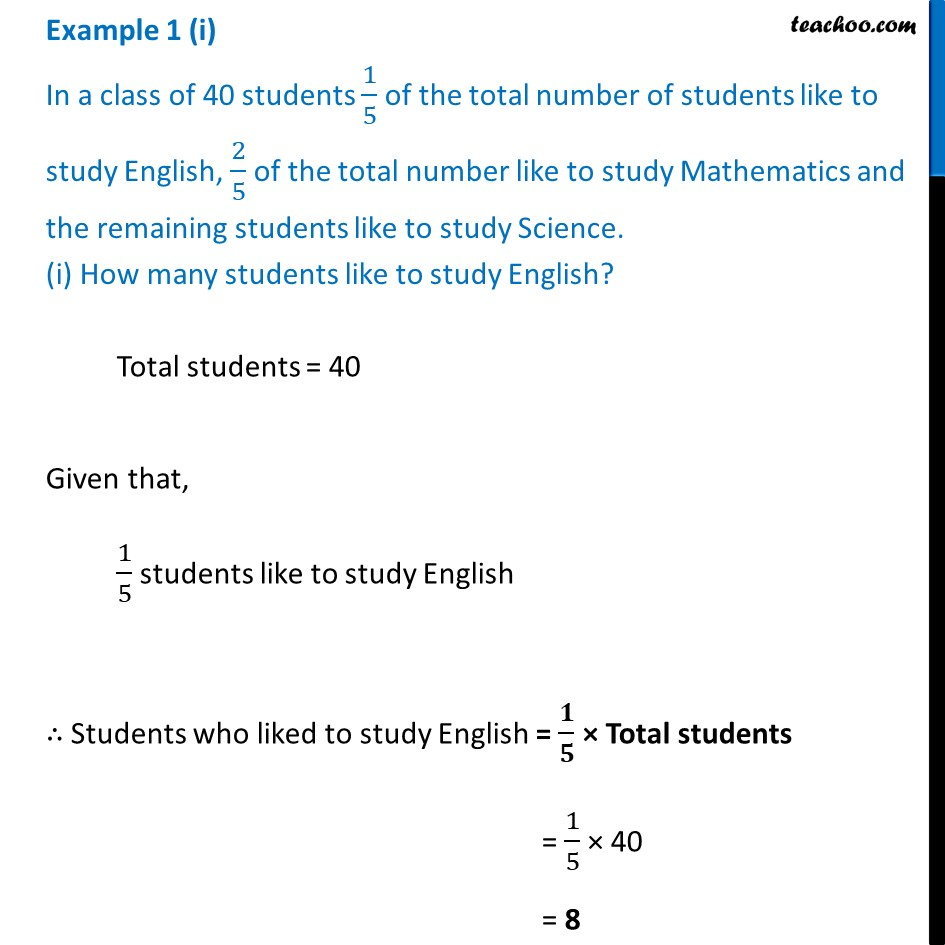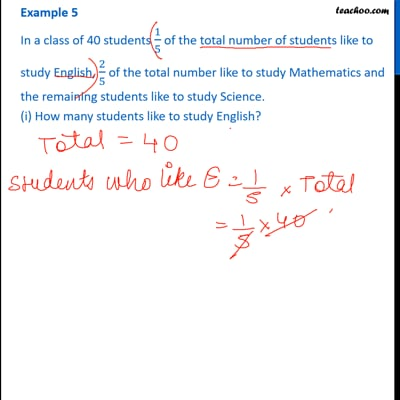This video is only available for Teachoo black users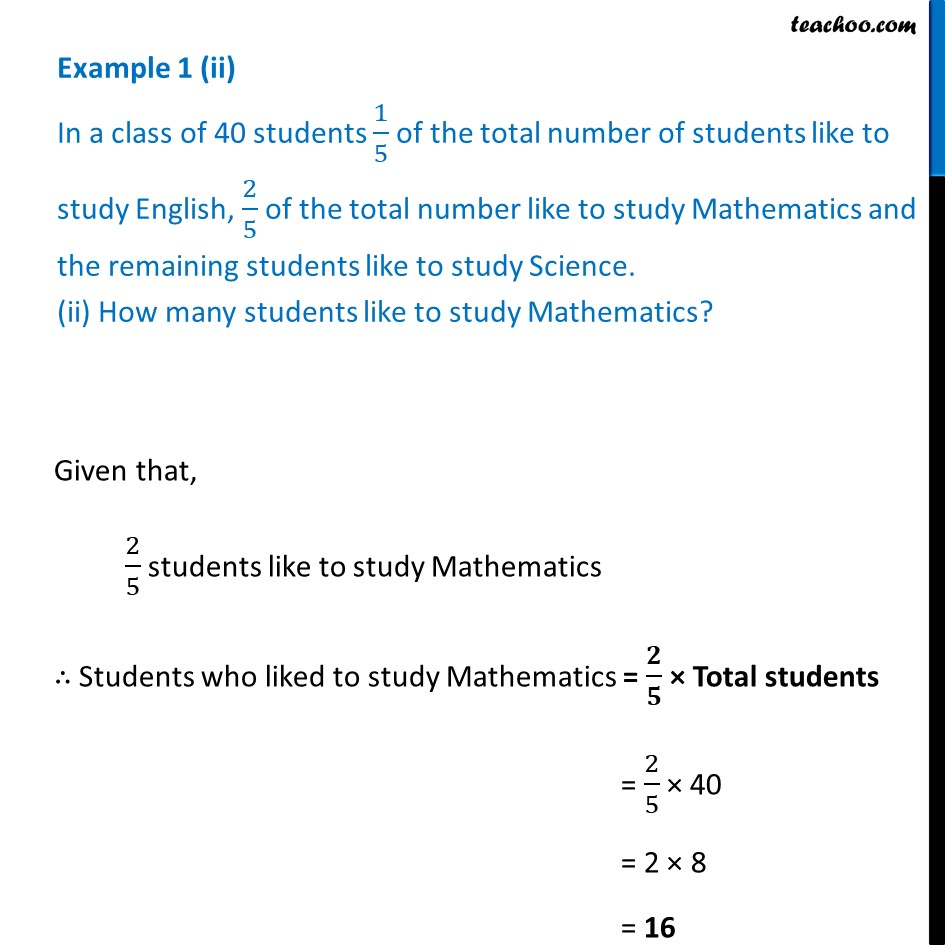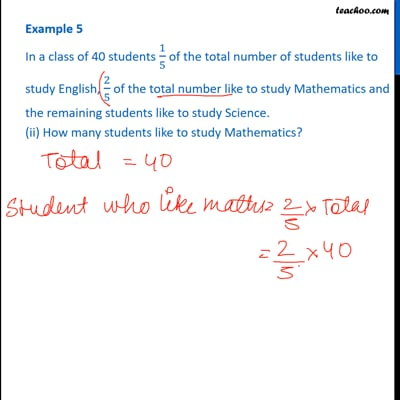This video is only available for Teachoo black users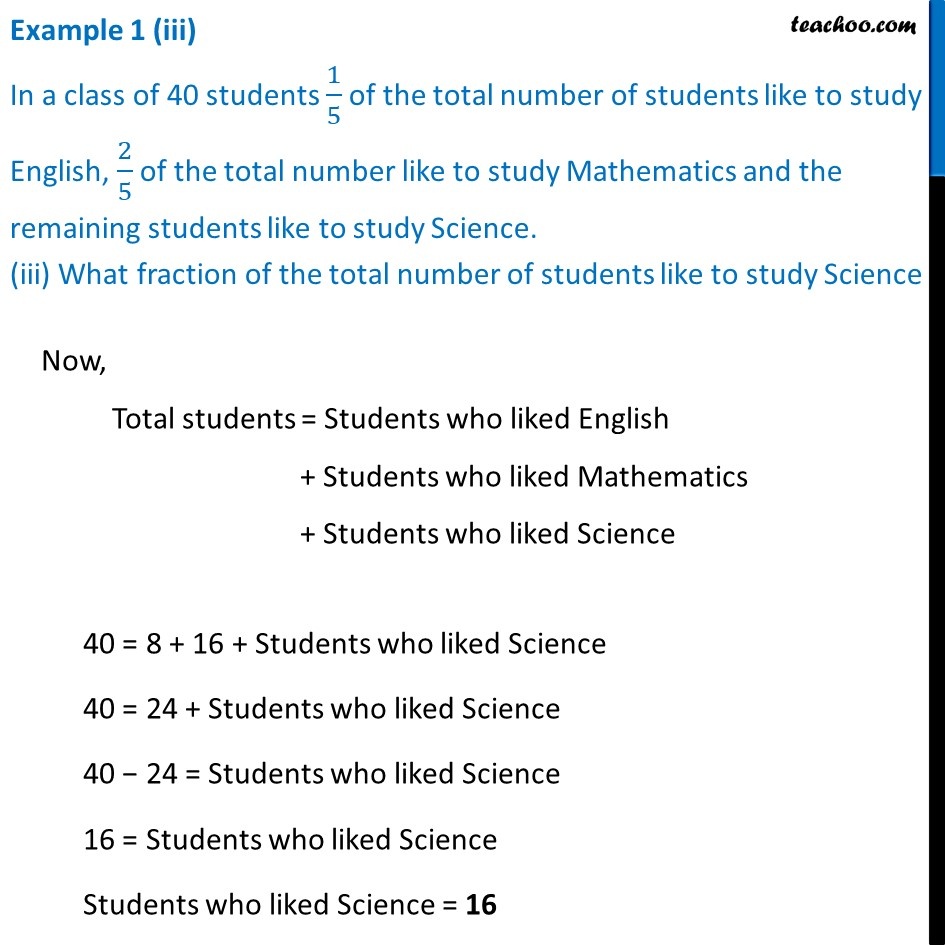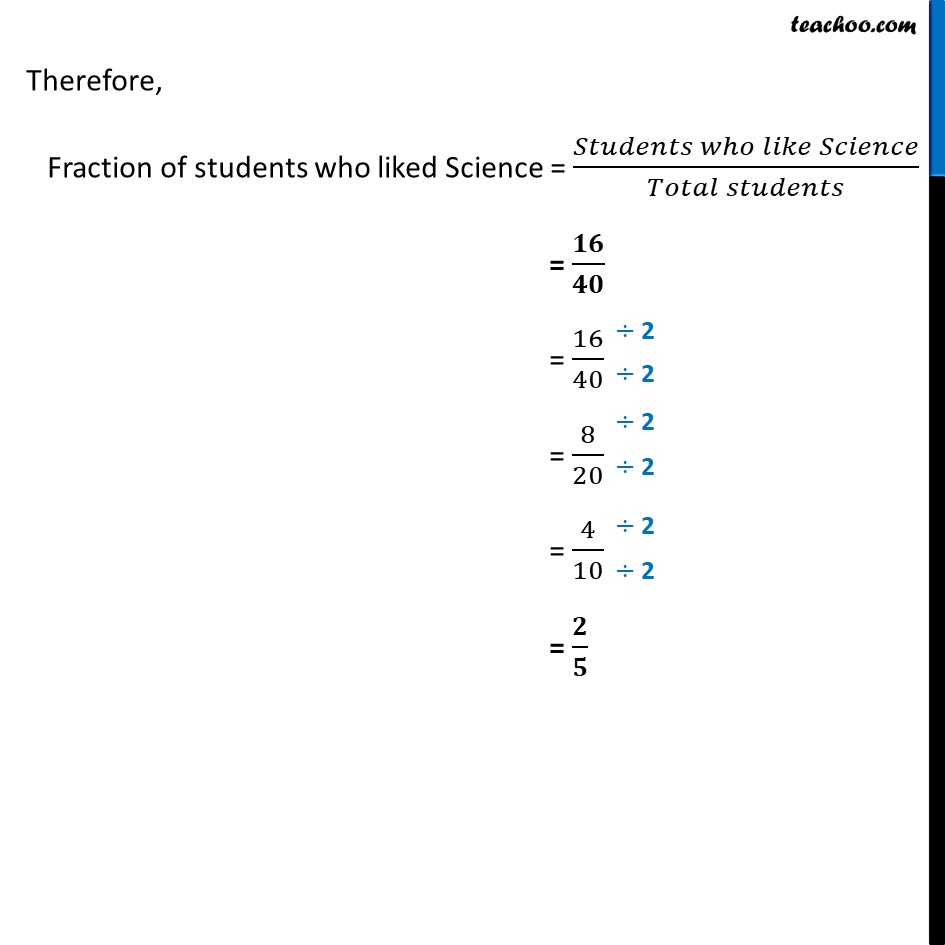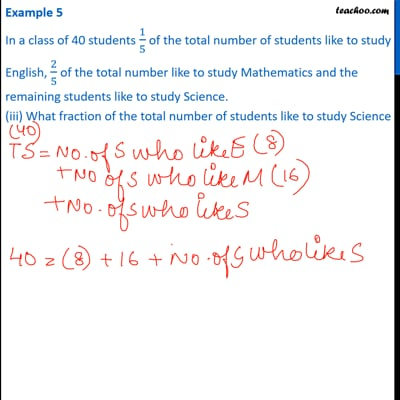This video is only available for Teachoo black users

Learn in your speed, with individual attention - Teachoo Maths 1-on-1 Class

### Transcript

Example 1 (i) In a class of 40 students 1/5 of the total number of students like to study English, 2/5 of the total number like to study Mathematics and the remaining students like to study Science. (i) How many students like to study English? Total students = 40 Given that, 1/5 students like to study English ∴ Students who liked to study English = 𝟏/𝟓 × Total students = 1/5 × 40 = 8 Example 1 (ii) In a class of 40 students 1/5 of the total number of students like to study English, 2/5 of the total number like to study Mathematics and the remaining students like to study Science. (ii) How many students like to study Mathematics? Given that, 2/5 students like to study Mathematics ∴ Students who liked to study Mathematics = 𝟐/𝟓 × Total students = 2/5 × 40 = 2 × 8 = 16 Example 1 (iii) In a class of 40 students 1/5 of the total number of students like to study English, 2/5 of the total number like to study Mathematics and the remaining students like to study Science. (iii) What fraction of the total number of students like to study ScienceNow, Total students = Students who liked English + Students who liked Mathematics + Students who liked Science 40 = 8 + 16 + Students who liked Science 40 = 24 + Students who liked Science 40 − 24 = Students who liked Science 16 = Students who liked Science Students who liked Science = 16 Therefore, Fraction of students who liked Science = (𝑆𝑡𝑢𝑑𝑒𝑛𝑡𝑠 𝑤ℎ𝑜 𝑙𝑖𝑘𝑒 𝑆𝑐𝑖𝑒𝑛𝑐𝑒)/(𝑇𝑜𝑡𝑎𝑙 𝑠𝑡𝑢𝑑𝑒𝑛𝑡𝑠) = 𝟏𝟔/𝟒𝟎 = 16/40 = 8/20 = 4/10 = 𝟐/𝟓